# Linear-fractional function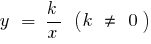## Properties of linear-fractional functions

1. Scope
2.3. Multiple value
4.5. Parity, odd parity
6. A function is odd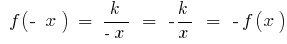, so its graph is symmetric relative to the origin

7. The point of intersection with the axes
8. Because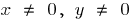, the points of intersection with the coordinate axes no

9. Continuity and differentiability
10. A function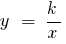is continuous at every point of its region of definition and is derived11. The increase and decrease of
12. when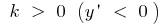a function is decreasing on each interval

when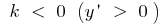a function is increasing on each interval

13. The graph of the function14. when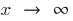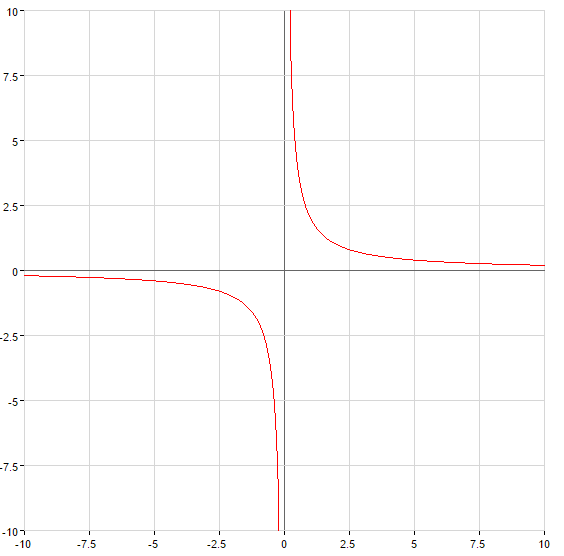Tags:
Chapter:
Versions in other languages:
Share with friends: